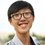# What do you know from this 2+2=5 video?

My friend asked me what is the meaning of this video. It states that only "genius mathematicians" can understand. Well, I am not a genius so I don't know what is it talking about. Personally, I don't think that this is a video that expresses some profound thinkings. However, here is the link.

Hope you all can give some comments!Note by Christopher Boo
7 years ago

This discussion board is a place to discuss our Daily Challenges and the math and science related to those challenges. Explanations are more than just a solution — they should explain the steps and thinking strategies that you used to obtain the solution. Comments should further the discussion of math and science.

When posting on Brilliant:

• Use the emojis to react to an explanation, whether you're congratulating a job well done , or just really confused .
• Ask specific questions about the challenge or the steps in somebody's explanation. Well-posed questions can add a lot to the discussion, but posting "I don't understand!" doesn't help anyone.
• Try to contribute something new to the discussion, whether it is an extension, generalization or other idea related to the challenge.
• Stay on topic — we're all here to learn more about math and science, not to hear about your favorite get-rich-quick scheme or current world events.

MarkdownAppears as
*italics* or _italics_ italics
**bold** or __bold__ bold
- bulleted- list
• bulleted
• list
1. numbered2. list
1. numbered
2. list
Note: you must add a full line of space before and after lists for them to show up correctly
paragraph 1paragraph 2

paragraph 1

paragraph 2

[example link](https://brilliant.org)example link
> This is a quote
This is a quote
    # I indented these lines
# 4 spaces, and now they show
# up as a code block.

print "hello world"
# I indented these lines
# 4 spaces, and now they show
# up as a code block.

print "hello world"
MathAppears as
Remember to wrap math in $$ ... $$ or $ ... $ to ensure proper formatting.
2 \times 3 $2 \times 3$
2^{34} $2^{34}$
a_{i-1} $a_{i-1}$
\frac{2}{3} $\frac{2}{3}$
\sqrt{2} $\sqrt{2}$
\sum_{i=1}^3 $\sum_{i=1}^3$
\sin \theta $\sin \theta$
\boxed{123} $\boxed{123}$

## Comments

Sort by:

Top Newest

Welcome to my sixth grade classroom, Foundations of Algebra! :D

- 7 years ago

Log in to reply

Nice video Christopher...!!!:)

- 7 years ago

Log in to reply

My dad taught me this when I was 8

$-20 = -20$

$= 16 - 36 = 25 - 45$

$= 16 - 36 + \frac{81}{4} = 25 - 45 + \frac{81}{4}$

$= 4^{2} - 2 \times 4 \times \frac{9}{2} + ( \frac{9}{2} )^{2} = 5^{2} - 2 \times 5 \times \frac{9}{2} + ( \frac{9}{2} )^{2}$

$= a^{2} - 2ab + b^{2} = a^{2} - 2ab + b^{2}$

$= (a-b)^{2} = (a-b)^{2}$

$= ( 4 - \frac{9}{2} )^{2} = (5 - \frac{9}{2} )^{2}$

$= ( 2 + 2 - \frac{9}{2} )^{2} = (5 - \frac{9}{2} )^{2}$

$\implies 2 + 2 - \frac{9}{2} = 5 - \frac{9}{2}$

$\implies 2 + 2 = \boxed{5}$

P.S. Of course it is incorrect ;) There is a very minute trick

- 7 years ago

Log in to reply

You took same variable ( a ) on both sides but with different value

- 6 years, 8 months ago

Log in to reply

It can be said that 2+2=5 but how can u say that if the squares of two numbers is equal than the numbers are also equal...for example, 4=4 but root 4 is not equal to root 4...as it can be +2 as well as -2...good explanation though Muzzafar...I was surprised for a moment...haha!!

- 7 years ago

Log in to reply

Actually it is

$( 4 - \frac{9}{2} )^{2} = ( 5 - \frac{9}{2} )^{2}$

$= ( - \frac{1}{2} )^{2} = (\frac{1}{2} )^{2}$

$= \frac{1}{4} = \frac{1}{4}$

- 7 years ago

Log in to reply

Moreover, u took letter 'a' as one variable but it has two different values which can't be true...One side a=4 and the other side a=5....

- 7 years ago

Log in to reply

That is why I told

There is a mistake :P ;)

- 7 years ago

Log in to reply

Thanks!

- 7 years ago

Log in to reply

So THAT's what O'Brien was trying to explain to Winston Smith. Four fingers is indeed FIVE.

- 7 years ago

Log in to reply

Yep it is

- 7 years ago

Log in to reply

This is from George Orwell's "1984", where Winston Smith, under torture and interrogation, is forced to say that party apparatchik O'Brien is holding up 5 fingers, when he knows he's only holding up 4 fingers. I don't think it takes a "genius mathematician" to understand how ideologies tend to force people into thinking and saying only certain proscribed things. Even though "1984" is supposedly a futuristic tale, information and thought control goes back thousands of years, as means of maintaining political power.

What's probably true is that "genius mathematicians" are adverse to rote or standardized thinking, which is a major problem today in American schools pretending to be teaching "math".

- 7 years ago

Log in to reply

Thanks for your explanation!

- 7 years ago

Log in to reply

×

Problem Loading...

Note Loading...

Set Loading...